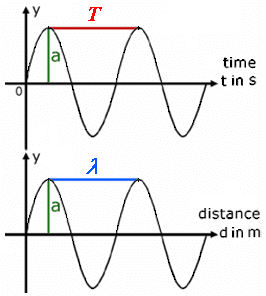# Help understanding about wavelength

anhnha
Hi,
I want to ask one question about wavelength. Here is a picture defining wavelength.
To get a sinusoidal wave in space, we have to use a sinusoidal voltage source at transmitter, right?
I mean if there is a sinusoidal voltage source at the transmitter to create a sinusoidal wave in space.## Answers and Replies

Gold Member
Yes, where T is the period of the wave, f=1/T is the frequency, and lambda is the wavelength.

Note that wavelength x frequency = speed of propagation for the wave. This is the phase velocity.

anhnha
Thank you, I wanted to know that to confirm my understanding about how wave propagating in space. For example,
there is a sinusoidal voltage source at transmitter and speed of propagation for the wave is v (m/s), the direction in which the wave moves is z.
Say, at t=0, the voltage at the source is 2V then at t=1s, at the point A that is v(m) away from the source in z direction, the voltage will be 2V, right?

Gold Member
If you are looking at an oscilloscope your phase will repeat in time steps of T=period of the wave=1/f.

Not sure what distance you are interested in here ...

anhnha
I don't understand why the waveform in all wavelength are all sinusoidal. What shape of wave in space if the signal generated at transmitter is not sinusoidal? And then how we can definition wavelength in this case?

thegreenlaser
I don't understand why the waveform in all wavelength are all sinusoidal. What shape of wave in space if the signal generated at transmitter is not sinusoidal? And then how we can definition wavelength in this case?

You could define terms like wavelength for a lot of non-sinusoidal waves, however there's a reason we usually focus on sinusoids. Fourier series/transform theory tells us that we can take any arbitrary wave and write it as a sum of sinusoidal waves. For example, a 2 Hz triangle wave can be written as a sum of 2 Hz, 4 Hz, 6 Hz, 8 Hz, etc. sinusoidal waves.

Rather than analyzing any arbitrary waveform (which may be messy and hard to understand), the easiest approach for a lot of problems is to break up an arbitrary non-sinusoidal waveform into sinusoidal components, analyze those components individually, and then add everything back up to get your result. It sounds like more work, but if you take a course on signal processing you'll quickly see the advantage. So basically, rather than study every kind of possible wave (assigning them wavelengths and what-not), we study sinusoids in great detail because we know that once we understand sinusoids, we can use that knowledge to understand a wide range of other types of waves.

Edit: by the way, you're right that a non-sinusoidal source would produce a non-sinusoidal wave. Your voice, for example, is a non-sinusoidal sound wave. However, like I explained above, it's usually much easier to describe your complicated vocal signal by breaking it down into sinusoidal waves rather than trying to analyze it directly.

Gold Member
Any wave has a period; you simply note when the wave repeats itself ...

But the simplest waveforms are sinusoids; you can build any wave from a collection of sinusoids - though it may take be a very large number, and sharp edges won't reproduce exactly.